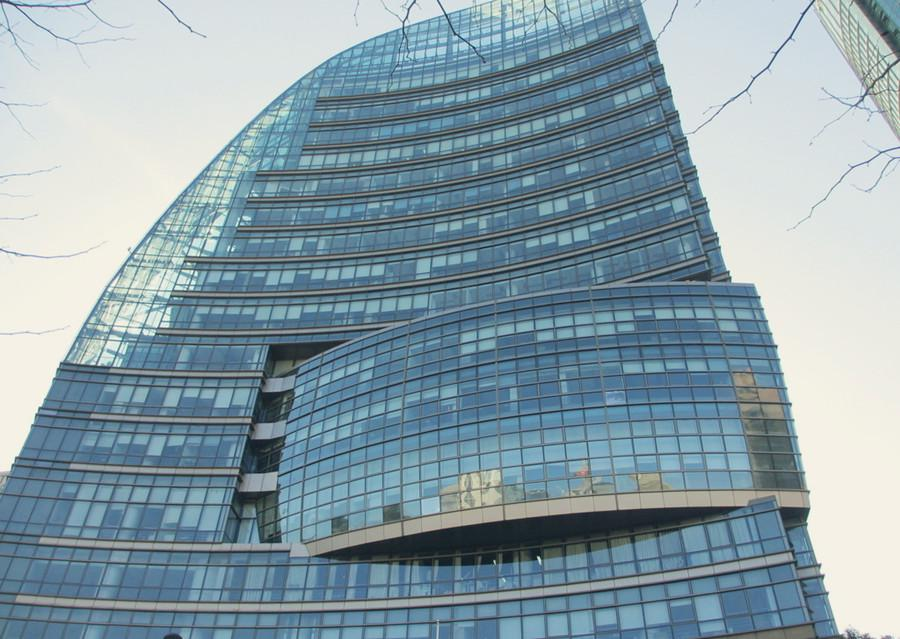# What is SC?What is the shading coefficient?

A measure of the ability of a window or skylight to transmit solar heat.

One of the important data for glass low e coating.

The shading coefficient is expressed as a number without units between 0 and 1.

The lower a window’s solar heat gain coefficient or shading coefficient,

the less solar heat it transmits, and the greater is its shading ability.

The solar factor (total transmittance) of a glass configuration is relative

to that of 3mm clear float glass (0.87) and is used as a performance comparison.

The lower the shading coefficient number, the lower the amount of solar heat transmitted.

The short wave shading coefficient is the direct transmittance (T) of the glass as a factor

of the solar factor or total transmittance (g or TT) of 3mm clear float glass (T ¸ 0.87).

The long wave-shading coefficient is the internally re-radiated energy that the glass has absorbed as glass.

It is determined by subtracting the direct transmittance from the solar factor (total transmittance)

of the subject glass and then dividing by the solar factor ( total transmittance)

of 3mm clear float glass (g-T ¸ 0.87).

It is typically used to describe the solar heat transmittance properties of glass, but has also been used for other translucent and transparent materials.

Solar transmittance is important for determining the solar heat gain into an enclosed space during sunny conditions. Solar heat gain can be beneficial in the winter, as it reduces the need for heating, but in the summer it can cause overheating.

The total solar heat transmittance is equal to the solar heat that is transmitted through the material directly, plus the solar heat that is absorbed by the material and then re-emitted into the enclosed space.

Shading coefficients can be measured using an illuminated hot box under simulated summer and winter conditions, and from these values, solar heat gain under a range of different conditions may be predicted using known data about solar heat gain through standard clear float glass. This enables the behaviour of translucent or transparent materials to be predicted under different environmental conditions without having to measure the angular optical properties of every individual material.

Total shading coefficients (TSC) can be broken down into short-wave shading coefficients (SWSC) and long-wave shading coefficients (LWSC).

Manufacturers are now moving towards the use of solar heat gain coefficients (SHGC) or window solar factors (g-values) rather than shading coefficients. These represent the fraction of incident solar radiation transmitted by a window, expressed as a number between 1 and 0, where 1 indicates the maximum possible solar heat gain, and zero, no solar heat gain.

In very approximate terms, the solar heat gain coefficient is equal to the shading coefficient x 0.87.